PRINTABLE FOR KIDS

XII (12) HSC

XI (11) FYJC
X (10) SSC

### Refraction Of Light Class 10th Science And Technology Part 1 MHB Solution

##### Class 10th Science And Technology Part 1 MHB Solution

###### Exercise
Question 1.

Fill in the blanks and Explain the completed sentences.

a. Refractive index depends on the ............. of light.

b. The change in ................ of light rays while going from one medium to another is called refraction.

(a) Refractive index depends on the velocity of light.

Explanation: The velocity of light is different in different medium. Speed of light is more in rarer medium as compared to denser medium. The medium in which speed of light is less, higher is the refractive index of that medium.

(b) The change in direction of light rays while going from one medium to another is called refraction.

Explanation: When a ray of light passes from one medium to another it changes its direction as speed of light is different in different medium. This phenomenon of light is called refraction.

Question 2.

Prove the following statements.

If the angle of incidence and angle of emergence of a light ray falling on a glass slab are i and e respectively, prove that, i = e.

i. Take a rectangular glass slab PQRS as shown in below figure.

ii. Draw an incident ray AO and a normal NN’. Measure the angle of incidence i.

iii. As light ray travels from air to glass i.e. rarer to denser medium it bends towards the normal. So refracted ray OB bend towards normal.

iv. Again light ray passes from glass to air at surface SR so emergent ray BC bends away from normal N1N1’. Measure the angle e.

v. We will find that angle of incidence i = angle of emergence e. We will also observed that incident ray and emergent ray are parallel to each other since angle of incidence i = angle of emergence e.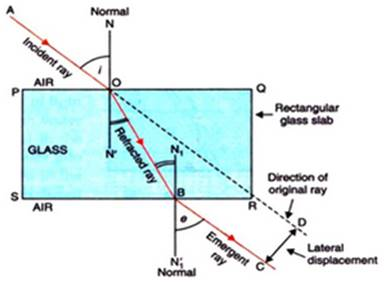Question 3.

Prove the following statements.

A rainbow is the combined effect of the refraction, dispersion, and total internal reflection of light.

Rainbow is a natural spectrum appearing in the sky after a rain shower. It is formed due to combination of phenomenon like refraction, dispersion and total internal reflection of light. Water droplets act like small prisms which refract and disperse the incident sunlight. Then they reflect it internally and finally again refract it to form rainbow in the sky.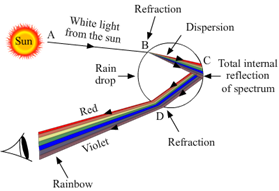Question 4.

Mark the correct answer in the following questions.

What is the reason for the twinkling of stars?
A. Explosions occurring in stars from time to time

B. Absorption of light in the earth’s atmosphere

C. Motion of stars

D. Changing refractive index of the atmospheric gases

Stars are point source of light very far from us. Light rays from stars passes through various layers of atmosphere whose refractive index goes on changing due to mobility of air, temperature and various gases present in air. So light suffer refraction when reaches to our eye and we see apparent position of star. Due to continuous change in refractive index of air sometimes star appear to us as bright or dim which appears to us as twinkling of star.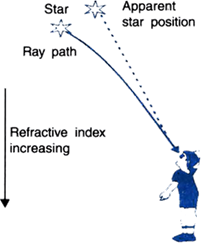Question 5.

Mark the correct answer in the following questions.

We can see the Sun even when it is little below the horizon because of
A. Reflection of light

B. Refraction of light

C. Dispersion of light

D. Absorption of light

During sunrise when sun is little below horizon the light rays move through different refractive indices of air and get bends towards the normal undergoing refraction so we see the apparent position of the sun S’. Thus even though the sun is below the horizon we can see the sunrise.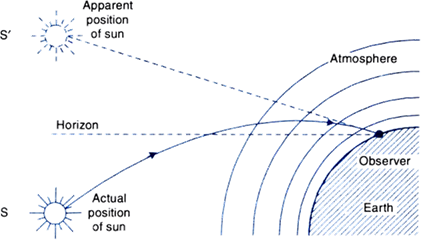Question 6.

Mark the correct answer in the following questions.

If the refractive index of glass with respect to air is 3/2, what is the refractive index of air with respect to glass?
A.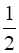B. 3

C.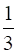D.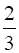Since refractive index of medium 2 with respect to medium 1 = 1/refractive index of medium 1 with respect to refractive index of medium 2. So if refractive index of glass with respect to air is 3/2 so refractive index of air with respect to glass =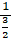=Question 7.

Solve the following examples.

If the speed of light in a medium is 1.5 x 108 m/s, what is the absolute refractive index of the medium?

Absolute refractive index of medium is with respect to air.

So absolute refractive index of medium μ =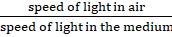Speed of light in air = 3 x 108 m/s

Speed of light in the given medium = 1.5 x 108 m/s

Absolute refractive index of medium μ =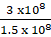So μ = 2

Question 8.

Solve the following examples.

If the absolute refractive indices of glass and water are 3/2 and 4/3 respectively, what is the refractive index of glass with respect to water?

Refractive Index of glass = 3/2

Refractive index of water = 4/3

So refractive index of glass with respect to water =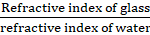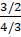= 9/8

So refractive index of glass with respect to water = 9/8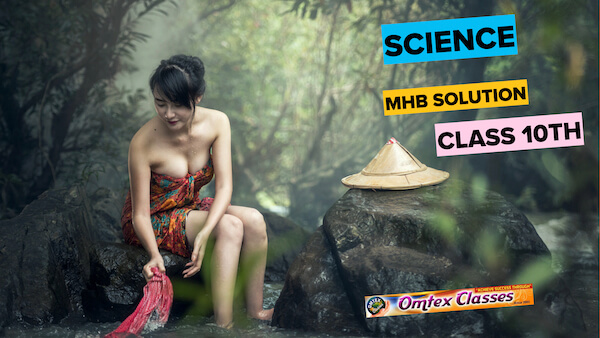## PDF FILE TO YOUR EMAIL IMMEDIATELY PURCHASE NOTES & PAPER SOLUTION. @ Rs. 50/- each (GST extra)

SUBJECTS

HINDI ENTIRE PAPER SOLUTION

MARATHI PAPER SOLUTION
SSC MATHS I PAPER SOLUTION
SSC MATHS II PAPER SOLUTION
SSC SCIENCE I PAPER SOLUTION
SSC SCIENCE II PAPER SOLUTION
SSC ENGLISH PAPER SOLUTION
SSC & HSC ENGLISH WRITING SKILL
HSC ACCOUNTS NOTES
HSC OCM NOTES
HSC ECONOMICS NOTES
HSC SECRETARIAL PRACTICE NOTES

2019 Board Paper Solution

HSC ENGLISH SET A 2019 21st February, 2019

HSC ENGLISH SET B 2019 21st February, 2019

HSC ENGLISH SET C 2019 21st February, 2019

HSC ENGLISH SET D 2019 21st February, 2019

SECRETARIAL PRACTICE (S.P) 2019 25th February, 2019

HSC XII PHYSICS 2019 25th February, 2019

CHEMISTRY XII HSC SOLUTION 27th, February, 2019

OCM PAPER SOLUTION 2019 27th, February, 2019

HSC MATHS PAPER SOLUTION COMMERCE, 2nd March, 2019

HSC MATHS PAPER SOLUTION SCIENCE 2nd, March, 2019

SSC ENGLISH STD 10 5TH MARCH, 2019.

HSC XII ACCOUNTS 2019 6th March, 2019

HSC XII BIOLOGY 2019 6TH March, 2019

HSC XII ECONOMICS 9Th March 2019

SSC Maths I March 2019 Solution 10th Standard11th, March, 2019

SSC MATHS II MARCH 2019 SOLUTION 10TH STD.13th March, 2019

SSC SCIENCE I MARCH 2019 SOLUTION 10TH STD. 15th March, 2019.

SSC SCIENCE II MARCH 2019 SOLUTION 10TH STD. 18th March, 2019.

SSC SOCIAL SCIENCE I MARCH 2019 SOLUTION20th March, 2019

SSC SOCIAL SCIENCE II MARCH 2019 SOLUTION, 22nd March, 2019

XII CBSE - BOARD - MARCH - 2019 ENGLISH - QP + SOLUTIONS, 2nd March, 2019

HSC Maharashtra Board Papers 2020

(Std 12th English Medium)

HSC ECONOMICS MARCH 2020

HSC OCM MARCH 2020

HSC ACCOUNTS MARCH 2020

HSC S.P. MARCH 2020

HSC ENGLISH MARCH 2020

HSC HINDI MARCH 2020

HSC MARATHI MARCH 2020

HSC MATHS MARCH 2020

SSC Maharashtra Board Papers 2020

(Std 10th English Medium)

English MARCH 2020

HindI MARCH 2020

Hindi (Composite) MARCH 2020

Marathi MARCH 2020

Mathematics (Paper 1) MARCH 2020

Mathematics (Paper 2) MARCH 2020

Sanskrit MARCH 2020

Important-formula

THANKS